Like   Tweet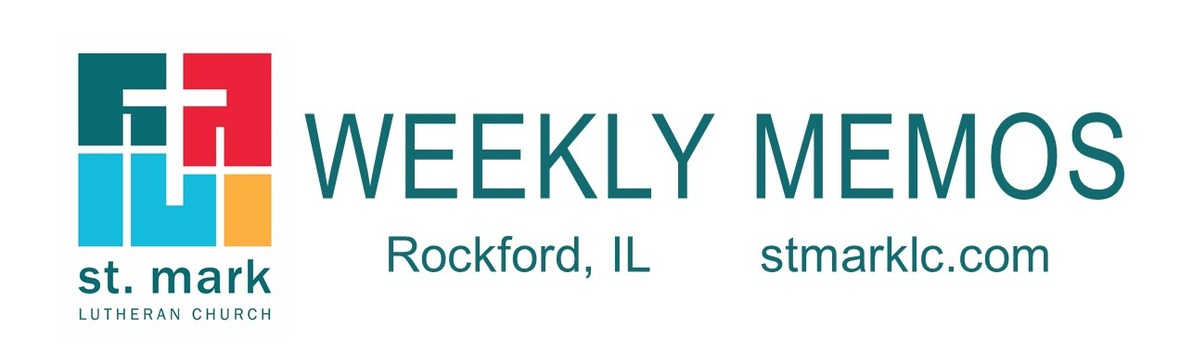## Pastoral Reflection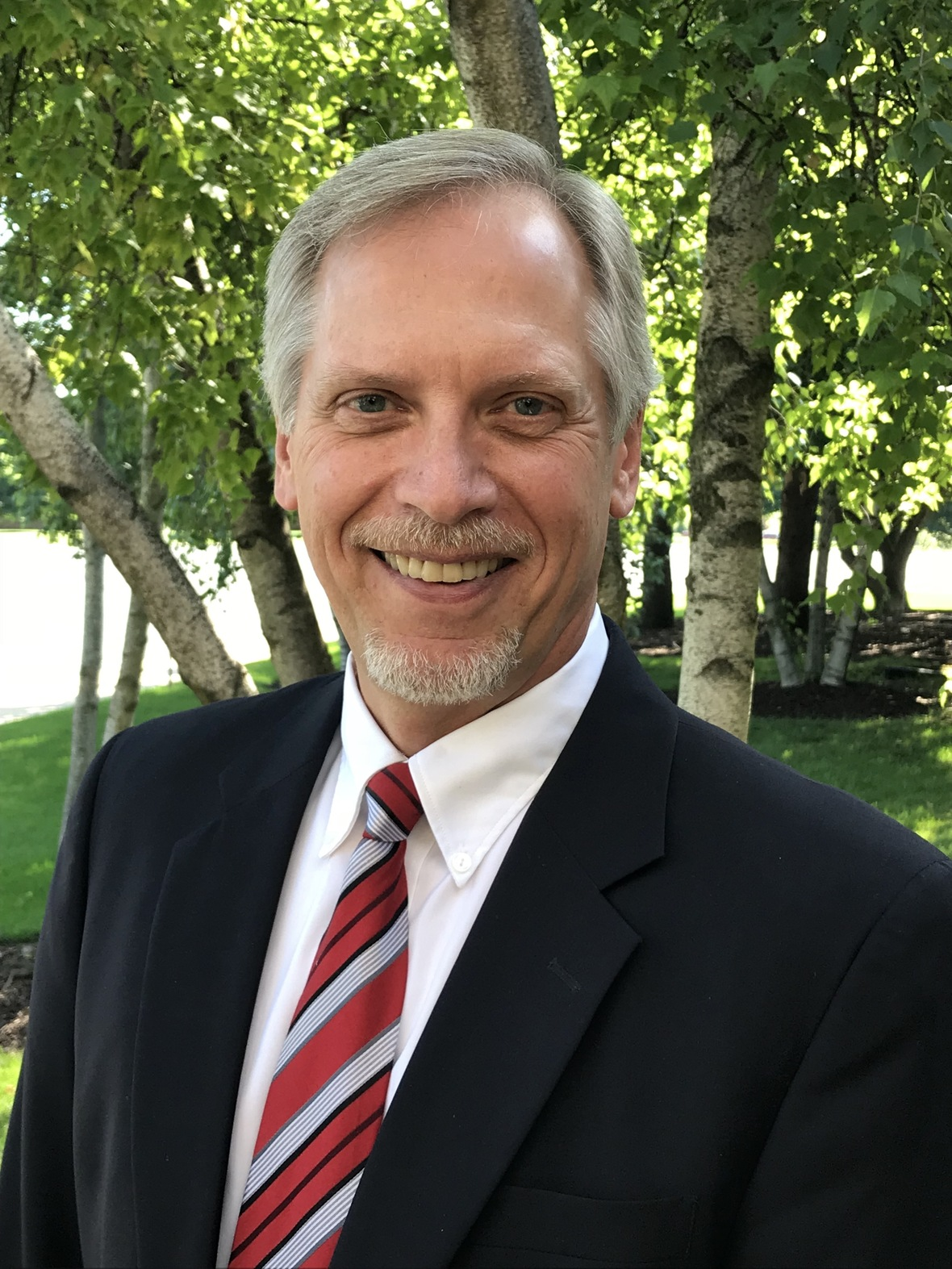Welcome, Wendy Iversen and Karen Osolin,
as New Office Staff!

Pastor Mark Hagen

As you know, we are blessed with a multitude of talented church staff members. Read more.

 table div table+table+table+table div table{width:100%;padding:0}table div table+table+table+table div table img{width:96.23%;padding:0;float:none}table div table+table+table+table div table td{width:100%;padding:0 1.88% 18px}/* styles */## Sunday, May 12

8:00 A.M. Worship
9:30 A.M. Worship
9:30 A.M. Sunday School Awards & Teacher Recognition
11:00 A.M. Worship

## Monday, May 13

9:00 A.M. Classic Chorale Rehearses
6:30 P.M. Emotions Anonymous

## Tuesday, May 14

10:00 A.M. Staff Meeting
11:00 A.M. St. Mark Duplicate Bridge
1:00 P.M. First Tuesday Book Club

## Wednesday, May 15

9:00 A.M. Men's Bible Study
9:30 A.M. St. Mark Ringers
5:30 P.M. Good News Bearers
6:15 P.M. Bible Study
6:30 P.M. Confirmation

## Thursday, May 16

7:30 A.M. Panera Book Study
9:00 A.M. Cherry Valley Bridge Group
10:15 A.M. Primetimers to WhitePines Theater
1:00 P.M. Sew and Sews
6:30 P.M. Emotions Anonymous
6:30 P.M. Diakonia
7:00 P.M. Praise Band Rehearses

## Friday, May 17

7:30 A.M. St. Mark Men's Breakfast at Denny's

## Sunday, May 19

8:00 A.M. Worship
9:30 A.M. Worship
11:00 A.M. Worship

 table div table+table+table+table+table+table+table+table div table{width:100%;padding:0}table div table+table+table+table+table+table+table+table div table img{width:96.23%;padding:0;float:none}table div table+table+table+table+table+table+table+table div table td{width:100%;padding:0 1.88% 18px}/* styles */## News and Events

 table div table+table+table+table+table+table+table+table+table+table div table{width:100%;padding:0}table div table+table+table+table+table+table+table+table+table+table div table img{width:96.23%;padding:0;float:none}table div table+table+table+table+table+table+table+table+table+table div table td{width:100%;padding:0 1.88% 18px}/* styles */Family Kite Flying Saturday!
Wind Swept Saturday, May 11, we are hosting a family Kite Flying event at 10:00 A.M. Join us at the Saturn Park for a morning of kite flying and field clean-up. We will celebrate spring and help to make the neighborhood more beautiful! Saturn Park is located at the corner of Garrett Lane and Trainer Road, across from the fire station behind Target. Kites will be provided.

 table div table+table+table+table+table+table+table+table+table+table+table+table div table{width:100%;padding:0}table div table+table+table+table+table+table+table+table+table+table+table+table div table img{width:96.23%;padding:0;float:none}table div table+table+table+table+table+table+table+table+table+table+table+table div table td{width:100%;padding:0 1.88% 18px}/* styles */Registration is now open for Vacation Bible School 2019
Volunteer for VBS too at the link below!

To Mars and Beyond: Explore Where God’s Power Can Take You!

Our amazing space adventures will take place from 9 am-noon on June 10-14. VBS is open to elementary-age students and includes daily snacks, crafts, science, games, music, Bible stories, and more! Register in person at St. Mark or online at myvbs.org/stmarklc.
Let’s explore God’s universe together!

 table div table+table+table+table+table+table+table+table+table+table+table+table+table+table div table{width:100%;padding:0}table div table+table+table+table+table+table+table+table+table+table+table+table+table+table div table img{width:96.23%;padding:0;float:none}table div table+table+table+table+table+table+table+table+table+table+table+table+table+table div table td{width:100%;padding:0 1.88% 18px}/* styles */Mulch “moving” party this Saturday!
Join us between 9:00-11:00 A.M. at the St. Mark playground. We’ve got wheelbarrows, shovels, and even buckets!
We need some helpers to move this mulch! Many hands make light work.

 table div table+table+table+table+table+table+table+table+table+table+table+table+table+table+table+table div table{width:100%;padding:0}table div table+table+table+table+table+table+table+table+table+table+table+table+table+table+table+table div table img{width:96.23%;padding:0;float:none}table div table+table+table+table+table+table+table+table+table+table+table+table+table+table+table+table div table td{width:100%;padding:0 1.88% 18px}/* styles */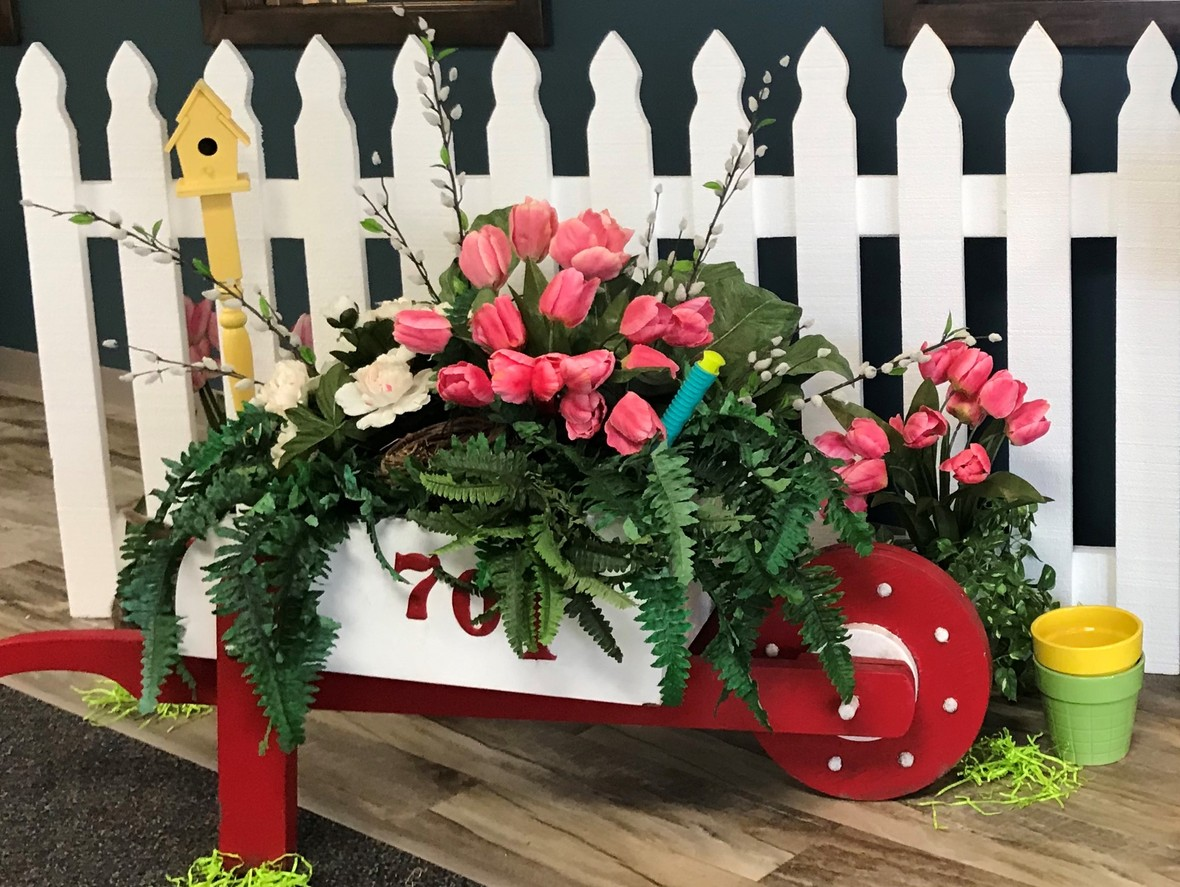We had such a lovely time. Thank you for your feedback. We have reviewed the “check marks” and have results of the most popular ideas for possible future events.
Yoga, Zumba, Tai Chi, Wine Tasting, healthy cooking, living with chronic disease, meditation & mindfulness, travel, nutrition, beginning Bible study, crafting, painting, jewelry making, floral arranging, bird feeders, herb & flower garden. Watch for details in future Memo’s!

 table div table+table+table+table+table+table+table+table+table+table+table+table+table+table+table+table+table+table div table{width:100%;padding:0}table div table+table+table+table+table+table+table+table+table+table+table+table+table+table+table+table+table+table div table img{width:96.23%;padding:0;float:none}table div table+table+table+table+table+table+table+table+table+table+table+table+table+table+table+table+table+table div table td{width:100%;padding:0 1.88% 18px}/* styles */Wednesday Morning Bible Studies
Wednesday morning women’s Bible study has completed their year of study and fellowship as of today that would be great. Also, the Bible study will be meeting on May 29th at 9:30 at Stockholm Inn to wrap up the year and give a welcome to summer! We will resume in the Fall.

Wednesday morning men's Bible study will complete their year at Stockholm Inn on May 15 at 9:00 a.m. They will resume in the Fall.

 table div table+table+table+table+table+table+table+table+table+table+table+table+table+table+table+table+table+table+table+table div table{width:100%;padding:0}table div table+table+table+table+table+table+table+table+table+table+table+table+table+table+table+table+table+table+table+table div table img{width:96.23%;padding:0;float:none}table div table+table+table+table+table+table+table+table+table+table+table+table+table+table+table+table+table+table+table+table div table td{width:100%;padding:0 1.88% 18px}/* styles */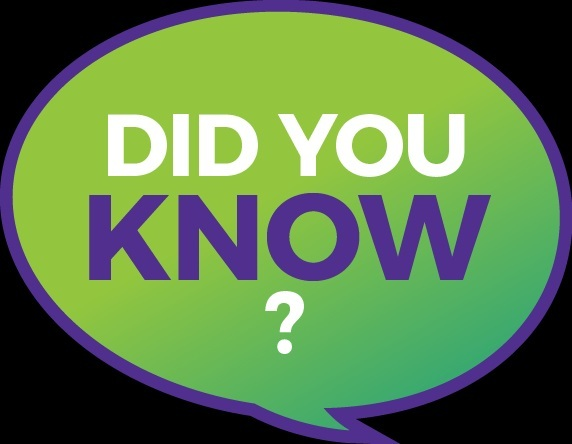Miss a Sunday at St. Mark? Did you know that you can watch the sermons online? Click here to watch.

 table div table+table+table+table+table+table+table+table+table+table+table+table+table+table+table+table+table+table+table+table+table+table div table{width:100%;padding:0}table div table+table+table+table+table+table+table+table+table+table+table+table+table+table+table+table+table+table+table+table+table+table div table img{width:96.23%;padding:0;float:none}table div table+table+table+table+table+table+table+table+table+table+table+table+table+table+table+table+table+table+table+table+table+table div table td{width:100%;padding:0 1.88% 18px}/* styles */Laundry Love Night
On Wednesday, May 15th, St. Mark will sponsor Laundry Love’s monthly open laundry night. In addition to seeking donations the Sunday before, we will also provide the volunteers for the evening. This busy night begins at 5:30 p.m. and ends roughly between 7:30 and 8:00 p.m. after the last client has been served. Volunteers will assist clients with soap and quarters and have plenty of time to talk with those who are there. It is truly a rewarding experience. If you want to participate, please contact Pastor Chad prmckenna@stmarklc.com Space is limited! Laundry Love happens at Charles Street Laundromat (2125 Charles St.) and is a ministry of Rockford Area Lutheran Ministries.

 table div table+table+table+table+table+table+table+table+table+table+table+table+table+table+table+table+table+table+table+table+table+table+table+table div table{width:100%;padding:0}table div table+table+table+table+table+table+table+table+table+table+table+table+table+table+table+table+table+table+table+table+table+table+table+table div table img{width:96.23%;padding:0;float:none}table div table+table+table+table+table+table+table+table+table+table+table+table+table+table+table+table+table+table+table+table+table+table+table+table div table td{width:100%;padding:0 1.88% 18px}/* styles */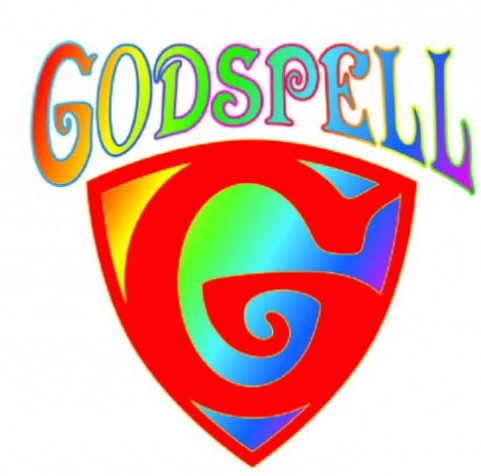Christian Youth Theater - Godspell will be at the Coronado Performing Art Center (307 Salem St. Rockton) Friday, May 17, @7:00 P.M., May 18, 2 & 6:00 P.M. and Sunday, May 19th at 2:00 P.M.
St. Mark Youth Group will be attending the Sunday afternoon performance. (see Melinda Alekna, Karen Anderson, or Kellie Champion for more information)

For tickets call 847-516-2298 or go to CYTCHICAGO.org

 table div table+table+table+table+table+table+table+table+table+table+table+table+table+table+table+table+table+table+table+table+table+table+table+table+table+table div table{width:100%;padding:0}table div table+table+table+table+table+table+table+table+table+table+table+table+table+table+table+table+table+table+table+table+table+table+table+table+table+table div table img{width:96.23%;padding:0;float:none}table div table+table+table+table+table+table+table+table+table+table+table+table+table+table+table+table+table+table+table+table+table+table+table+table+table+table div table td{width:100%;padding:0 1.88% 18px}/* styles */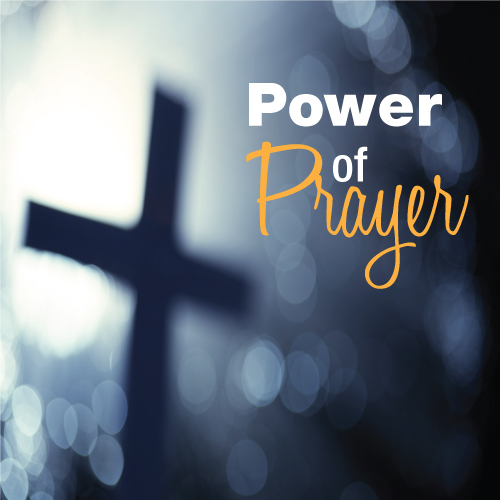St. Mark Prayer Chain
Would you like to be a part of the St. Mark prayer chain? Prayer requests come into the church office weekly. As we receive prayer requests we would send out an e-mail and ask that you pray daily for the persons mentioned in the requests. All requests are to remain confidential. Please e-mail melinda@stmarklc.com if you would like to participate in the prayer chain. “Be anxious for nothing, but in everything by prayer and supplication, with thanksgiving, let your requests be made known to God.” Colossians 4:2.

 table div table+table+table+table+table+table+table+table+table+table+table+table+table+table+table+table+table+table+table+table+table+table+table+table+table+table+table+table div table{width:100%;padding:0}table div table+table+table+table+table+table+table+table+table+table+table+table+table+table+table+table+table+table+table+table+table+table+table+table+table+table+table+table div table img{width:96.23%;padding:0;float:none}table div table+table+table+table+table+table+table+table+table+table+table+table+table+table+table+table+table+table+table+table+table+table+table+table+table+table+table+table div table td{width:100%;padding:0 1.88% 18px}/* styles */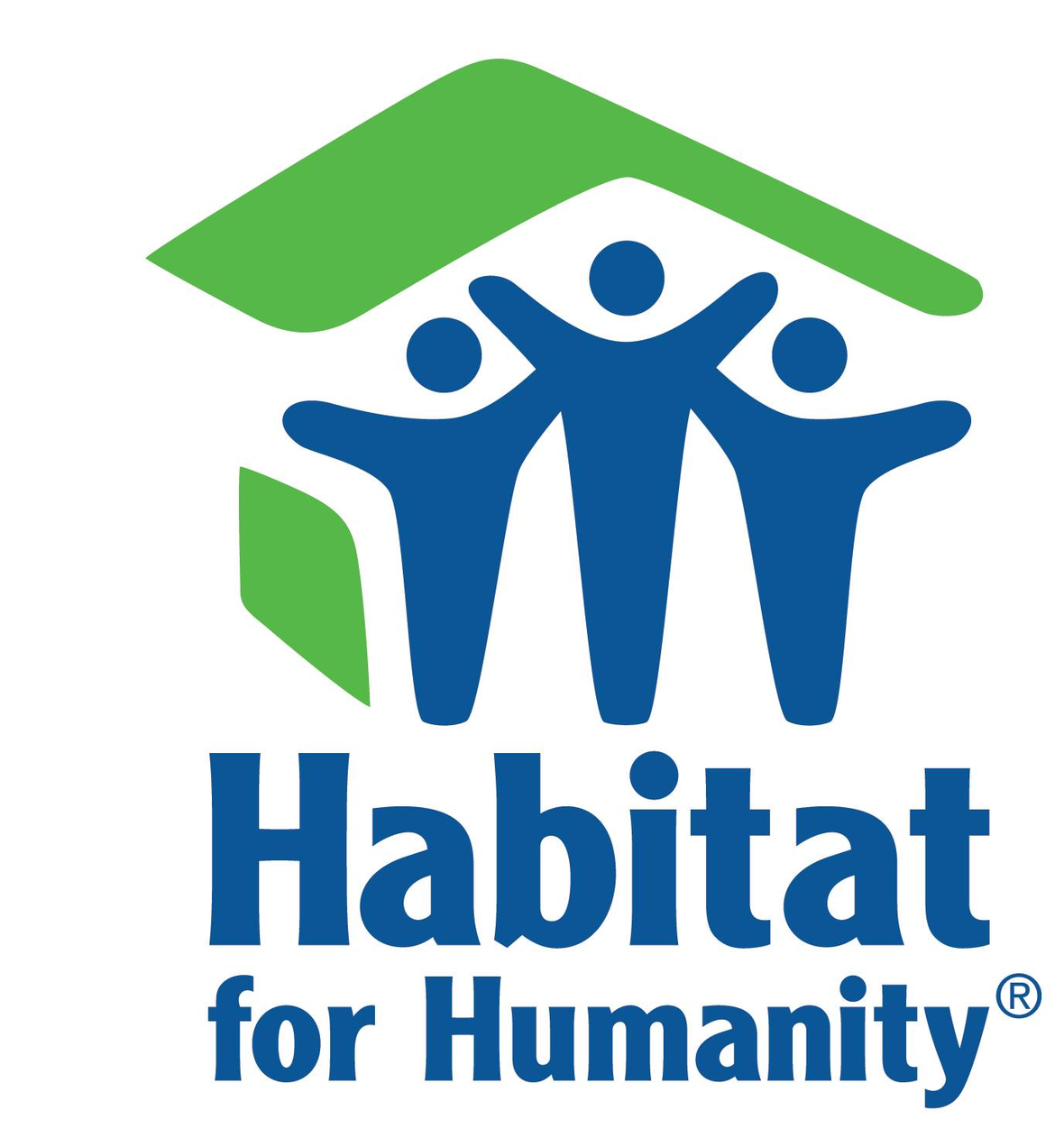Habitat for Humanity Framing Days
Our local Habitat chapter has kicked off the build season on Saturday, May 4th, with their first of four framing days. No matter how handy you are with a hammer, your help is always welcome! To sign up, please go to rockfordhabitat.org/events.

 table div table+table+table+table+table+table+table+table+table+table+table+table+table+table+table+table+table+table+table+table+table+table+table+table+table+table+table+table+table+table div table{width:100%;padding:0}table div table+table+table+table+table+table+table+table+table+table+table+table+table+table+table+table+table+table+table+table+table+table+table+table+table+table+table+table+table+table div table img{width:96.23%;padding:0;float:none}table div table+table+table+table+table+table+table+table+table+table+table+table+table+table+table+table+table+table+table+table+table+table+table+table+table+table+table+table+table+table div table td{width:100%;padding:0 1.88% 18px}/* styles */## Youth MinistrySummer Youth Trip to the Apostle Islands is June 24-28. We have a couple of seats left in the van. We hope to sign-up a couple more youth in March. If you have questions or are interested please email or call Melinda Alekna melinda@stmarklc.com or 815-871-0390.

 table div table+table+table+table+table+table+table+table+table+table+table+table+table+table+table+table+table+table+table+table+table+table+table+table+table+table+table+table+table+table+table+table+table div table{width:100%;padding:0}table div table+table+table+table+table+table+table+table+table+table+table+table+table+table+table+table+table+table+table+table+table+table+table+table+table+table+table+table+table+table+table+table+table div table img{width:96.23%;padding:0;float:none}table div table+table+table+table+table+table+table+table+table+table+table+table+table+table+table+table+table+table+table+table+table+table+table+table+table+table+table+table+table+table+table+table+table div table td{width:100%;padding:0 1.88% 18px}/* styles */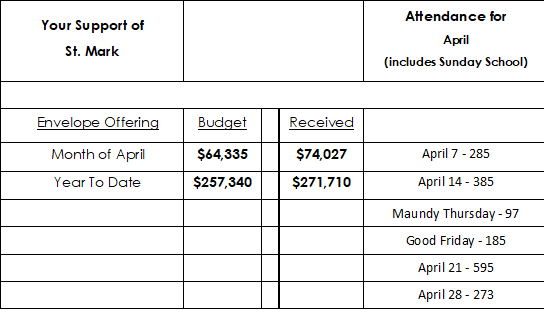table div table+table+table+table+table+table+table+table+table+table+table+table+table+table+table+table+table+table+table+table+table+table+table+table+table+table+table+table+table+table+table+table+table+table+table div table{width:100%;padding:0}table div table+table+table+table+table+table+table+table+table+table+table+table+table+table+table+table+table+table+table+table+table+table+table+table+table+table+table+table+table+table+table+table+table+table+table div table img{width:96.23%;padding:0;float:none}table div table+table+table+table+table+table+table+table+table+table+table+table+table+table+table+table+table+table+table+table+table+table+table+table+table+table+table+table+table+table+table+table+table+table+table div table td{width:100%;padding:0 1.88% 18px}/* styles */## Our St. Mark members:

Deb Lindley, Kim Edgcomb, David Young, Russ Oellig, Linda Swanson, Pam Cottrill, Keith Haggestad, Naomi Hagen.

## Our condolences to:

Paul and Mary Panther upon the death of Paul's mother, JoAnne Panther.

## Family and friends of St. Mark members:

Chuck Blomgren, Earl Karau, Darold Beekmann, Ann Eck, Clay Heinrich, Denise Nichols, Mary Graham, Doug Nelson, Barbara, Heckart, Tom Wolfe, Kim Menke, Noah and Ellie Adams, Norma Comstock, Scott Jones, Ethan Whitney, Carolyn King, Tom Severson, Katie Butera, Ashton Henley, Nathan Gyllin.

## Those serving in the military and their families:

Bailey Panjkovich, Xander Gehrke, Jim Tammen, Brandon Ballenger, Taylor Eversole, Dean Barron.

 table div table+table+table+table+table+table+table+table+table+table+table+table+table+table+table+table+table+table+table+table+table+table+table+table+table+table+table+table+table+table+table+table+table+table+table+table+table+table div table{width:100%;padding:0}table div table+table+table+table+table+table+table+table+table+table+table+table+table+table+table+table+table+table+table+table+table+table+table+table+table+table+table+table+table+table+table+table+table+table+table+table+table+table div table img{width:96.23%;padding:0;float:none}table div table+table+table+table+table+table+table+table+table+table+table+table+table+table+table+table+table+table+table+table+table+table+table+table+table+table+table+table+table+table+table+table+table+table+table+table+table+table div table td{width:100%;padding:0 1.88% 18px}/* styles *//* styles */ Office Hours Monday - Friday, 8:00 A.M. - 4:00 P.M. Telephone: 815-398-3557 Publications St. Mark Memos, the weekly e-newsletter, is distributed each Thursday. The deadline for submission is Monday by 4:00 P.M. Please click to send submissions. Sermons Online Miss a Sunday at St. Mark? Please visit our website and watch the sermons online. Please click to watch.